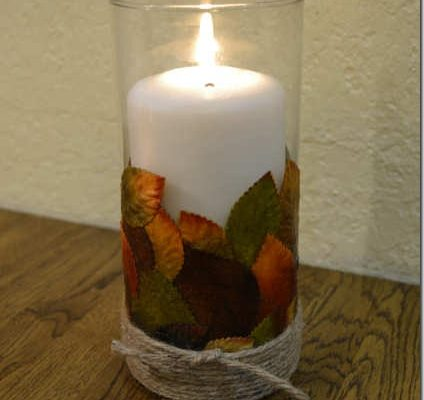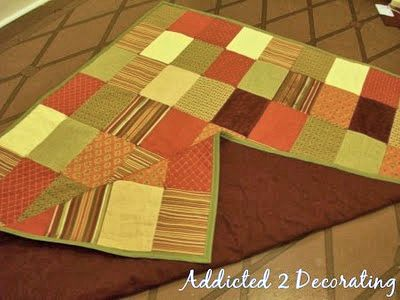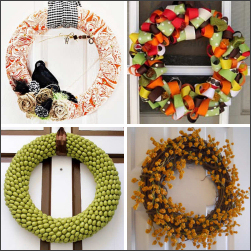Last Updated on January 8, 2016 by Kristi Linauer

Centerpieces for your autumn and Thanksgiving table don’t have to be expensive or elaborate, as Jenna from SAS Interiors shows us.  She used a free tree branch to make a tea light candleholder.  Brilliant!

To see how Jenna made this quick and free centerpiece, visit SAS Interiors.

``` ```
``` On October 12, 2011 • By Kristi Linauer 2 You Might Also Like...Decoupaged Fall Leaf Vase/Candle Holder October 3, 2011Simple Quilted Patchwork Throw April 6, 2010DIY Fall Wreath Roundup September 21, 2011 Previous Post DIY Light Fixture For My Hallway Next Post How To Decorate A Fall Mantel 2 Comments Leave a Comment Name * Email * Website Δdocument.getElementById( "ak_js_1" ).setAttribute( "value", ( new Date() ).getTime() ); This site uses Akismet to reduce spam. Learn how your comment data is processed. Reply To This Comment ↓ terra October 12, 2011 at 11:48 am great )) so warm Reply To This Comment ↓ Jenna, SAS Interiors October 12, 2011 at 6:22 pm Thanks for sharing this!  Much appreciated and glad you like it!~Jenna, SAS Interiors ```
``` ```
``` ```
``` ```
``` Well, hello there! I’m Kristi…I'm a DIY fanatic remodeling my way through our 1948 fixer upper.More About Kristi Addicted 2 Decorating is a participant in the Amazon Services LLC Associates Program, an affiliate advertising program designed to provide a means for sites to earn advertising fees by advertising and linking to Amazon.com. ```
``` ```
``` addicted2decorating I was concerned about how well these FLOR carpet tOur home gym is finally finished! And yes, even aThe new Flor carpet tiles are installed in the homIt’s hard to believe that when we bought this hoToday on the blog, I shared five home improvementI still need to finish some projects on the frontLoad More... Follow on Instagram Facebook Twitter Instagram Pinterest Youtube Copyright 2007-2022 - Kristi Linauer, LLC. All Rights Reserved. Addicted 2 Decorating is a participant in the Amazon Services LLC Associates Program, an affiliate advertising program designed to provide a means for sites to earn advertising fees by advertising and linking to Amazon.com. Privacy Policy Terms and Conditions ```
``` ```
``` 'use strict';(function(){function B(c,b){function a(){this.constructor=c}if("function"!==typeof b&&null!==b)throw new TypeError("Class extends value "+String(b)+" is not a constructor or null");O(c,b);c.prototype=null===b?Object.create(b):(a.prototype=b.prototype,new a)}function R(c,b,a,d){var e=arguments.length,f=3>e?b:null===d?d=Object.getOwnPropertyDescriptor(b,a):d,g;if("object"===typeof Reflect&&"function"===typeof Reflect.decorate)f=Reflect.decorate(c,b,a,d);else for(var h=c.length-1;0<=h;h--)if(g= c[h])f=(3>e?g(f):3<e?g(b,a,f):g(b,a))||f;return 3<e&&f&&Object.defineProperty(b,a,f),f}function I(c,b){if("object"===typeof Reflect&&"function"===typeof Reflect.metadata)return Reflect.metadata(c,b)}function J(c){var b="function"===typeof Symbol&&Symbol.iterator,a=b&&c[b],d=0;if(a)return a.call(c);if(c&&"number"===typeof c.length)return{next:function(){c&&d>=c.length&&(c=void 0);return{value:c&&c[d++],done:!c}}};throw new TypeError(b?"Object is not iterable.":"Symbol.iterator is not defined.");}function p(c, b){var a="function"===typeof Symbol&&c[Symbol.iterator];if(!a)return c;c=a.call(c);var d,e=[];try{for(;(void 0===b||0<b--)&&!(d=c.next()).done;)e.push(d.value)}catch(g){var f={error:g}}finally{try{d&&!d.done&&(a=c["return"])&&a.call(c)}finally{if(f)throw f.error;}}return e}function w(c,b,a){if(a||2===arguments.length)for(var d=0,e=b.length,f;d<e;d++)!f&&d in b||(f||(f=Array.prototype.slice.call(b,0,d)),f[d]=b[d]);return c.concat(f||Array.prototype.slice.call(b))}window.adthriveCLS.buildDate="2023-03-30"; var t=function(c,b){return null==c||c!==c?b:c},ja=function(c){var b=c.clientWidth;getComputedStyle&&(c=getComputedStyle(c,null),b-=parseFloat(c.paddingLeft||"0")+parseFloat(c.paddingRight||"0"));return b},S=function(c){var b=c.offsetHeight,a=c.offsetWidth,d=c.getBoundingClientRect(),e=document.body,f=document.documentElement;c=Math.round(d.top+(window.pageYOffset||f.scrollTop||e.scrollTop)-(f.clientTop||e.clientTop||0));d=Math.round(d.left+(window.pageXOffset||f.scrollLeft||e.scrollLeft)-(f.clientLeft|| e.clientLeft||0));return{top:c,left:d,bottom:c+b,right:d+a,width:a,height:b}},T=function(){var c=navigator.userAgent,b=/Tablet|iPad|Playbook|Nook|webOS|Kindle|Android (?!.*Mobile).*Safari/i.test(c);return/Mobi|iP(hone|od)|Opera Mini/i.test(c)&&!b},O=function(c,b){O=Object.setPrototypeOf||{__proto__:[]}instanceof Array&&function(a,d){a.__proto__=d}||function(a,d){for(var e in d)Object.prototype.hasOwnProperty.call(d,e)&&(a[e]=d[e])};return O(c,b)},x=function(){x=Object.assign||function(c){for(var b, a=1,d=arguments.length;a<d;a++){b=arguments[a];for(var e in b)Object.prototype.hasOwnProperty.call(b,e)&&(c[e]=b[e])}return c};return x.apply(this,arguments)},ka=function(c){void 0===c&&(c=document);return(c===document?document.body:c).getBoundingClientRect().top},la=function(c){return c.includes(",")?c.split(","):[c]},ma=function(c){void 0===c&&(c=document);c=c.querySelectorAll("article");return 0===c.length?null:(c=Array.from(c).reduce(function(b,a){return a.offsetHeight>b.offsetHeight?a:b}))&& c.offsetHeight>1.5*window.innerHeight?c:null},na=function(c,b,a){void 0===a&&(a=document);var d=ma(a),e=d?[d]:[],f=[];c.forEach(function(h){var l=Array.from(a.querySelectorAll(h.elementSelector)).slice(0,h.skip);la(h.elementSelector).forEach(function(k){var u=a.querySelectorAll(k);k=function(r){var m=u[r];if(b.map.some(function(z){return z.el.isEqualNode(m)}))return"continue";(r=m&&m.parentElement)&&r!==document.body?e.push(r):e.push(m);-1===l.indexOf(m)&&f.push({dynamicAd:h,element:m})};for(var q= 0;q<u.length;q++)k(q)})});var g=ka(a);c=f.sort(function(h,l){return h.element.getBoundingClientRect().top-g-(l.element.getBoundingClientRect().top-g)});return[e,c]},oa=function(c,b,a){void 0===a&&(a=document);b=p(na(c,b,a),2);c=b;b=b;if(0===c.length)throw Error("No Main Content Elements Found");return[Array.from(c).reduce(function(d,e){return e.offsetHeight>d.offsetHeight?e:d})||document.body,b]},A;(function(c){c.ThirtyThreeAcross="33across";c.AppNexus="appnexus";c.Amazon="amazon";c.Brightroll= "brightroll";c.Colossus="colossus";c.ColossusServer="col_ss";c.Conversant="conversant";c.Concert="concert";c.Criteo="criteo";c.GumGum="gumgum";c.IndexExchange="ix";c.Kargo="kargo";c.KargoServer="krgo_ss";c.MediaGrid="grid";c.MediaGridVideo="gridvid";c.Nativo="nativo";c.OpenX="openx";c.OpenXServer="opnx_ss";c.Pubmatic="pubmatic";c.PubmaticServer="pubm_ss";c.ResetDigital="resetdigital";c.Roundel="roundel";c.Rubicon="rubicon";c.RubiconServer="rubi_ss";c.Sharethrough="sharethrough";c.Spotx="spotx";c.Teads= "teads";c.Telaria="telaria";c.Triplelift="triplelift";c.TripleliftServer="tripl_ss";c.TTD="ttd";c.Undertone="undertone";c.UndertoneServer="under_ss";c.Unruly="unruly";c.YahooSSP="yahoossp";c.Verizon="verizon";c.Yieldmo="yieldmo"})(A||(A={}));var C;(function(c){c.Prebid="prebid";c.GAM="gam";c.Amazon="amazon";c.WebVitals="webvitals";c.Marmalade="marmalade";c.Floors="floors";c.CMP="cmp"})(C||(C={}));var U;(function(c){c.fpvNonLazy="fpvNonLazy";c.lazy="lazy";c.refresh="refresh";c.session="session";c.crossDomain= "crossdomain";c.highSequence="highsequence"})(U||(U={}));var V;(function(c){c.prebidLoad="prebidLoad";c.amazonLoad="amazonLoad";c.gptLoad="gptLoad"})(V||(V={}));var H;(function(c){c.Desktop="desktop";c.Mobile="mobile"})(H||(H={}));var D;(function(c){c.Video_Collapse_Autoplay_SoundOff="Video_Collapse_Autoplay_SoundOff";c.Video_Individual_Autoplay_SOff="Video_Individual_Autoplay_SOff";c.Video_Coll_SOff_Smartphone="Video_Coll_SOff_Smartphone";c.Video_In_Post_ClicktoPlay_SoundOn="Video_In-Post_ClicktoPlay_SoundOn"})(D|| (D={}));var W;(W||(W={})).None="none";var X;(function(c){c.WEIGHTEDCHOICE="weightedChoice";c.BERNOULLITRIAL="bernoulliTrial"})(X||(X={}));var Y;(function(c){c.INTEGER="integer";c.FLOAT="float";c.BOOLEAN="boolean";c.STRING="string"})(Y||(Y={}));var Z;(function(c){c.DESKTOP="desktop";c.MOBILE="mobile";c.ALL="all"})(Z||(Z={}));var E;(function(c){c.AdDensity="addensity";c.FooterCloseButton="footerclose";c.Interstitial="interstitial";c.RemoveVideoTitleWrapper="removevideotitlewrapper";c.StickyOutstream= "stickyoutstream";c.StickyOutstreamOnStickyPlayer="sospp";c.VideoAdvancePlaylistRelatedPlayer="videoadvanceplaylistrp";c.MobileStickyPlayerPosition="mspp"})(E||(E={}));var pa=function(c,b){var a=c.adDensityEnabled;c=c.adDensityLayout.pageOverrides.find(function(d){return!!document.querySelector(d.pageSelector)&&(d[b].onePerViewport||"number"===typeof d[b].adDensity)});return a?!c:!0};A=function(){function c(){this._timeOrigin=0}c.prototype.resetTimeOrigin=function(){this._timeOrigin=window.performance.now()}; c.prototype.now=function(){try{return Math.round(window.performance.now()-this._timeOrigin)}catch(b){return 0}};return c}();window.adthrive.windowPerformance=window.adthrive.windowPerformance||new A;A=window.adthrive.windowPerformance;var P=A.now.bind(A),Q=function(c){try{return{valid:!0,elements:document.querySelectorAll(c)}}catch(b){return x({valid:!1},b)}},aa=function(c){return""===c?{valid:!0}:Q(c)},n=new (function(){function c(){}c.prototype.info=function(b,a){for(var d=[],e=2;e<arguments.length;e++)d[e- 2]=arguments[e];this.call.apply(this,w([console.info,b,a],p(d),!1))};c.prototype.warn=function(b,a){for(var d=[],e=2;e<arguments.length;e++)d[e-2]=arguments[e];this.call.apply(this,w([console.warn,b,a],p(d),!1))};c.prototype.error=function(b,a){for(var d=[],e=2;e<arguments.length;e++)d[e-2]=arguments[e];this.call.apply(this,w([console.error,b,a],p(d),!1));this.sendErrorLogToCommandQueue.apply(this,w([b,a],p(d),!1))};c.prototype.event=function(b,a){for(var d,e=2;e<arguments.length;e++);"debug"===(null=== (d=window.adthriveCLS)||void 0===d?void 0:d.bucket)&&this.info(b,a)};c.prototype.sendErrorLogToCommandQueue=function(b,a){for(var d=[],e=2;e<arguments.length;e++)d[e-2]=arguments[e];window.adthrive=window.adthrive||{};window.adthrive.cmd=window.adthrive.cmd||[];window.adthrive.cmd.push(function(){void 0!==window.adthrive.logError&&"function"===typeof window.adthrive.logError&&window.adthrive.logError(b,a,d)}.bind(b,a,d))};c.prototype.call=function(b,a,d){for(var e=[],f=3;f<arguments.length;f++)e[f- 3]=arguments[f];f=["%c".concat(a,"::").concat(d," ")];var g=["color: #999; font-weight: bold;"];0<e.length&&"string"===typeof e&&f.push(e.shift());g.push.apply(g,w([],p(e),!1));try{Function.prototype.apply.call(b,console,w([f.join("")],p(g),!1))}catch(h){console.error(h)}};return c}()),qa=function(c){var b=c.reduce(function(a,d){return d.weight?d.weight+a:a},0);return 0<c.length&&c.every(function(a){var d=a.value;a=a.weight;return!(void 0===d||null===d||"number"===typeof d&&isNaN(d)||!a)})&&100=== b},ra=["siteId","siteName","adOptions","breakpoints","adUnits"],ba=function(){function c(){this._clsGlobalData=window.adthriveCLS}Object.defineProperty(c.prototype,"enabled",{get:function(){var b;if(b=!!this._clsGlobalData&&!!this._clsGlobalData.siteAds)a:{b=this._clsGlobalData.siteAds;var a=void 0;void 0===a&&(a=ra);if(b){for(var d=0;d<a.length;d++)if(!b[a[d]]){b=!1;break a}b=!0}else b=!1}return b},enumerable:!1,configurable:!0});Object.defineProperty(c.prototype,"error",{get:function(){return!(!this._clsGlobalData|| !this._clsGlobalData.error)},enumerable:!1,configurable:!0});Object.defineProperty(c.prototype,"siteAds",{get:function(){return this._clsGlobalData.siteAds},set:function(b){this._clsGlobalData.siteAds=b},enumerable:!1,configurable:!0});Object.defineProperty(c.prototype,"disableAds",{get:function(){return this._clsGlobalData.disableAds},set:function(b){this._clsGlobalData.disableAds=b},enumerable:!1,configurable:!0});Object.defineProperty(c.prototype,"enabledLocations",{get:function(){return this._clsGlobalData.enabledLocations}, set:function(b){this._clsGlobalData.enabledLocations=b},enumerable:!1,configurable:!0});Object.defineProperty(c.prototype,"injectedFromPlugin",{get:function(){return this._clsGlobalData.injectedFromPlugin},set:function(b){this._clsGlobalData.injectedFromPlugin=b},enumerable:!1,configurable:!0});Object.defineProperty(c.prototype,"injectedFromSiteAds",{get:function(){return this._clsGlobalData.injectedFromSiteAds},set:function(b){this._clsGlobalData.injectedFromSiteAds=b},enumerable:!1,configurable:!0}); c.prototype.overwriteInjectedSlots=function(b){this._clsGlobalData.injectedSlots=b};c.prototype.setInjectedSlots=function(b){this._clsGlobalData.injectedSlots=this._clsGlobalData.injectedSlots||[];this._clsGlobalData.injectedSlots.push(b)};Object.defineProperty(c.prototype,"injectedSlots",{get:function(){return this._clsGlobalData.injectedSlots},enumerable:!1,configurable:!0});c.prototype.setInjectedVideoSlots=function(b){this._clsGlobalData.injectedVideoSlots=this._clsGlobalData.injectedVideoSlots|| [];this._clsGlobalData.injectedVideoSlots.push(b)};Object.defineProperty(c.prototype,"injectedVideoSlots",{get:function(){return this._clsGlobalData.injectedVideoSlots},enumerable:!1,configurable:!0});c.prototype.setInjectedScripts=function(b){this._clsGlobalData.injectedScripts=this._clsGlobalData.injectedScripts||[];this._clsGlobalData.injectedScripts.push(b)};Object.defineProperty(c.prototype,"getInjectedScripts",{get:function(){return this._clsGlobalData.injectedScripts},enumerable:!1,configurable:!0}); c.prototype.setExperiment=function(b,a,d){void 0===d&&(d=!1);this._clsGlobalData.experiments=this._clsGlobalData.experiments||{};this._clsGlobalData.siteExperiments=this._clsGlobalData.siteExperiments||{};(d?this._clsGlobalData.siteExperiments:this._clsGlobalData.experiments)[b]=a};c.prototype.getExperiment=function(b,a){void 0===a&&(a=!1);return(a=a?this._clsGlobalData.siteExperiments:this._clsGlobalData.experiments)&&a[b]};c.prototype.setWeightedChoiceExperiment=function(b,a,d){void 0===d&&(d=!1); this._clsGlobalData.experimentsWeightedChoice=this._clsGlobalData.experimentsWeightedChoice||{};this._clsGlobalData.siteExperimentsWeightedChoice=this._clsGlobalData.siteExperimentsWeightedChoice||{};(d?this._clsGlobalData.siteExperimentsWeightedChoice:this._clsGlobalData.experimentsWeightedChoice)[b]=a};c.prototype.getWeightedChoiceExperiment=function(b,a){var d,e;void 0===a&&(a=!1);return(a=a?null===(d=this._clsGlobalData)||void 0===d?void 0:d.siteExperimentsWeightedChoice:null===(e=this._clsGlobalData)|| void 0===e?void 0:e.experimentsWeightedChoice)&&a[b]};Object.defineProperty(c.prototype,"branch",{get:function(){return this._clsGlobalData.branch},enumerable:!1,configurable:!0});Object.defineProperty(c.prototype,"bucket",{get:function(){return this._clsGlobalData.bucket},enumerable:!1,configurable:!0});Object.defineProperty(c.prototype,"videoDisabledFromPlugin",{get:function(){return this._clsGlobalData.videoDisabledFromPlugin},set:function(b){this._clsGlobalData.videoDisabledFromPlugin=b},enumerable:!1, configurable:!0});Object.defineProperty(c.prototype,"targetDensityLog",{get:function(){return this._clsGlobalData.targetDensityLog},set:function(b){this._clsGlobalData.targetDensityLog=b},enumerable:!1,configurable:!0});return c}(),sa=function(){function c(){}c.getScrollTop=function(){return(window.pageYOffset||document.documentElement.scrollTop)-(document.documentElement.clientTop||0)};c.shufflePlaylist=function(b){for(var a=b.length,d,e;0!==a;)e=Math.floor(Math.random()*b.length),--a,d=b[a],b[a]= b[e],b[e]=d;return b};c.isMobileLandscape=function(){return window.matchMedia("(orientation: landscape) and (max-height: 480px)").matches};c.playerViewable=function(b){b=b.getBoundingClientRect();return this.isMobileLandscape()?window.innerHeight>b.top+b.height/2&&0<b.top+b.height/2:window.innerHeight>b.top+b.height/2};c.playerVisible=function(b){b=b.getBoundingClientRect();return window.innerHeight>b.top};c.createQueryString=function(b){return Object.keys(b).map(function(a){return"".concat(a,"=").concat(b[a])}).join("&")}; c.createEncodedQueryString=function(b){return Object.keys(b).map(function(a){return"".concat(a,"=").concat(encodeURIComponent(b[a]))}).join("&")};c.setMobileLocation=function(b){b=b||"bottom-right";"top-left"===b?b="adthrive-collapse-top-left":"top-right"===b?b="adthrive-collapse-top-right":"bottom-left"===b?b="adthrive-collapse-bottom-left":"bottom-right"===b?b="adthrive-collapse-bottom-right":"top-center"===b&&(b=T()?"adthrive-collapse-top-center":"adthrive-collapse-bottom-right");return b};return c}(), ta=function(){return function(c){this._clsOptions=c;this.removeVideoTitleWrapper=t(this._clsOptions.siteAds.adOptions.removeVideoTitleWrapper,!1);c=this._clsOptions.siteAds.videoPlayers;this.footerSelector=t(c&&c.footerSelector,"");this.players=t(c&&c.players.map(function(b){b.mobileLocation=sa.setMobileLocation(b.mobileLocation);return b}),[]);this.contextualSettings=c&&c.contextual}}(),ua=function(){return function(c){this.contextualPlayerAdded=this.playlistPlayerAdded=this.mobileStickyPlayerOnPage= !1;this.footerSelector="";this.removeVideoTitleWrapper=!1;this.videoAdOptions=new ta(c);this.players=this.videoAdOptions.players;this.contextualSettings=this.videoAdOptions.contextualSettings;this.removeVideoTitleWrapper=this.videoAdOptions.removeVideoTitleWrapper;this.footerSelector=this.videoAdOptions.footerSelector}}();C=function(){return function(){}}();var ca=function(c){function b(a){var d=c.call(this)||this;d._probability=a;return d}B(b,c);b.prototype.get=function(){if(0>this._probability|| 1<this._probability)throw Error("Invalid probability: ".concat(this._probability));return Math.random()<this._probability};return b}(C);A=function(){function c(){this._clsOptions=new ba;this.shouldUseCoreExperimentsConfig=!1}c.prototype.setExperimentKey=function(b){void 0===b&&(b=!1);this._clsOptions.setExperiment(this.key,this.result,b)};return c}();var va=function(c){function b(){var a=c.call(this)||this;a._result=!1;a._choices=[{choice:!0},{choice:!1}];a.key="smhd100";a._result=a.run();a.setExperimentKey(); return a}B(b,c);Object.defineProperty(b.prototype,"result",{get:function(){return this._result},enumerable:!1,configurable:!0});b.prototype.run=function(){return(new ca(.1)).get()};return b}(A),wa=[[728,90],[300,250],[300,600],[320,50],[970,250],[160,600],[300,1050],[336,280],[970,90],[300,50],[320,100],[468,60],[250,250],[120,240],[1,1],[300,300],[552,334],[300,420],[728,250],[320,300],[300,390]],xa=new Map([["Footer",1],["Header",2],["Sidebar",3],["Content",4],["Recipe",5],["Sidebar_sticky",6], ["Below Post",7]]),ya=function(c){return wa.filter(function(b){b=p(b,2);var a=b,d=b;return c.some(function(e){e=p(e,2);var f=e;return a===e&&d===f})})},za=function(c,b){var a=new va;return b.filter(function(d){return void 0!==d.dynamic&&d.dynamic.enabled}).map(function(d){var e=d.location.replace(/\s+/g,"_");return{auctionPriority:xa.get(e)||8,location:e,sequence:t(d.sequence,1),sizes:ya(d.adSizes).filter(function(f){var g=d.location,h=p(f,2);f=h;h=h;return!("Footer"===g&&"phone"=== c&&320===f&&100===h||"Header"===g&&100<h&&a.result)}),devices:d.devices,pageSelector:t(d.dynamic.pageSelector,"").trim(),elementSelector:t(d.dynamic.elementSelector,"").trim(),position:t(d.dynamic.position,"beforebegin"),max:Math.floor(t(d.dynamic.max,0)),spacing:t(d.dynamic.spacing,0),skip:Math.floor(t(d.dynamic.skip,0)),every:Math.max(Math.floor(t(d.dynamic.every,1)),1),classNames:d.dynamic.classNames||[],sticky:"Footer"===d.location?!0:d.sticky,stickyOverlapSelector:t(d.stickyOverlapSelector,"").trim(), autosize:d.autosize,special:t(d.targeting,[]).filter(function(f){return"special"===f.key}).reduce(function(f,g){return f.concat.apply(f,w([],p(g.value),!1))},[]),lazy:t(d.dynamic.lazy,!1),lazyMax:t(d.dynamic.lazyMax,2),lazyMaxDefaulted:0===d.dynamic.lazyMax?!1:!d.dynamic.lazyMax}})},da=function(c,b){var a=ja(b),d=c.sticky&&"Sidebar"===c.location;return c.sizes.filter(function(e){var f=d?e<=window.innerHeight-100:!0;return(c.autosize?e<=a||320>=e:!0)&&f})},Aa=function(){return function(c){this.clsOptions= c;this.enabledLocations=["Below_Post","Content","Recipe","Sidebar"]}}(),Ba=function(c){var b=document.body;c="adthrive-device-".concat(c);if(!b.classList.contains(c))try{b.classList.add(c)}catch(a){n.error("BodyDeviceClassComponent","init",{message:a.message}),b="classList"in document.createElement("_"),n.error("BodyDeviceClassComponent","init.support",{support:b})}},Ca=function(c){if(c&&c.length){for(var b=0,a=0;a<c.length;a++){var d=Q(c[a]);if(d.valid){b=S(d.elements).height;break}}return b}}, Da=function(c,b){void 0===b&&(b=5);c='\n .adthrive-device-phone .adthrive-sticky-content {\n height: 450px !important;\n margin-bottom: 100px !important;\n }\n .adthrive-content.adthrive-sticky {\n position: -webkit-sticky;\n position: sticky !important;\n top: 42px !important;\n margin-top: 42px !important;\n }\n .adthrive-content.adthrive-sticky:after {\n content: "\u2014 Advertisement. Scroll down to continue. \u2014";\n font-size: 10pt;\n margin-top: 5px;\n margin-bottom: 5px;\n display:block;\n color: #888;\n }\n body.single .adthrive-content {\n position: relative;\n display: flex;\n flex-direction: column;\n justify-content: flex-start;\n align-items: center;\n min-height:'.concat(c? c:400,"px !important;\n margin: 10px 0 10px 0;\n background-color: #FAFAFA;\n padding-bottom:0px;\n }\n body.single .adthrive-content > div {\n flex-basis: unset;\n position: sticky !important;\n display: block;\n flex-direction: column;\n top: ").concat(5<b?b:5,"px;\n }\n ");b=void 0;void 0===b&&(b={});b=b.insertAt;if(c&&"undefined"!==typeof document){var a=document.head||document.getElementsByTagName("head"),d=document.createElement("style");d.type="text/css";"top"=== b?a.firstChild?a.insertBefore(d,a.firstChild):a.appendChild(d):a.appendChild(d);d.styleSheet?d.styleSheet.cssText=c:d.appendChild(document.createTextNode(c))}},ea=function(c){function b(a,d){void 0===a&&(a=[]);var e=c.call(this)||this;e._choices=a;e._default=d;return e}B(b,c);b.fromArray=function(a,d){return new b(a.map(function(e){e=p(e,2);return{choice:e,weight:e}}),d)};b.prototype.addChoice=function(a,d){this._choices.push({choice:a,weight:d})};b.prototype.get=function(){var a,d=100*Math.random(), e=0;try{for(var f=J(this._choices),g=f.next();!g.done;g=f.next()){var h=g.value,l=h.choice;e+=h.weight;if(e>=d)return l}}catch(u){var k={error:u}}finally{try{g&&!g.done&&(a=f.return)&&a.call(f)}finally{if(k)throw k.error;}}return this._default};Object.defineProperty(b.prototype,"totalWeight",{get:function(){return this._choices.reduce(function(a,d){return a+d.weight},0)},enumerable:!1,configurable:!0});return b}(C),Ea=function(c){for(var b=5381,a=c.length;a;)b=33*b^c.charCodeAt(--a);return b>>>0}, K=new (function(){function c(){var b=this;this.name="Session";this.disable=!1;this.removeSessionValue=function(a){window.localStorage.removeItem("adthrive_".concat(a.toLowerCase()))};this.getSessionValue=function(a,d,e){void 0===e&&(e=!1);if(b.disable)return null;try{var f=window.localStorage.getItem("adthrive_".concat(a.toLowerCase()));if(f){var g=JSON.parse(f),h=void 0!==d&&Date.now()-g.created>=d;if(g&&!h)return e&&b.setSessionValue(a,g.value),g.value}}catch(l){}return null};this.setSessionValue= function(a,d){try{var e={value:d,created:Date.now()};window.localStorage.setItem("adthrive_".concat(a.toLowerCase()),JSON.stringify(e))}catch(f){}};this.isValidABGroupSessionValue=function(a){return void 0!==a&&null!==a&&!("number"===typeof a&&isNaN(a))};this.getOrSetSessionValue=function(a,d,e,f,g){void 0===f&&(f=!0);e=b.getSessionValue(a,e,f);if(null!==e)return e;d=d();b.setSessionValue(a,d);g&&g(d);return d};this.getOrSetABGroupSessionValue=function(a,d,e,f,g){var h;void 0===f&&(f=!0);e=b.getSessionValue("abgroup", e,f);if(null!==e&&(f=e[a],b.isValidABGroupSessionValue(f)))return f;d=d();a=x(x({},e),(h={},h[a]=d,h));b.setSessionValue("abgroup",a);g&&g();return d}}c.prototype.init=function(){};return c}()),Fa=function(){return function(c,b,a){var d=a.value;d&&(a.value=function(){for(var e=this,f,g=[],h=0;h<arguments.length;h++)g[h]=arguments[h];h=Array.isArray(this._choices)?Ea(JSON.stringify(this._choices)).toString(16):null;var l=this.key?this.key.toLowerCase():"";h=h?"".concat(l,"_").concat(h):l;h=this.sessionStoragePrefix? "".concat(this.sessionStoragePrefix,"-").concat(h):h;!1===(null===(f=K.getSessionValue("branch"))||void 0===f?void 0:f.enabled)&&K.removeSessionValue(h);return K.getOrSetABGroupSessionValue(h,function(){return d.apply(e,g)},864E5)})}};C=function(c){function b(){var a=null!==c&&c.apply(this,arguments)||this;a._resultValidator=function(){return!0};return a}B(b,c);b.prototype._isValidResult=function(a){var d=this;return c.prototype._isValidResult.call(this,a,function(){return d._resultValidator(a)|| "control"===a})};b.prototype.run=function(){if(!this.enabled)return n.error("CLSWeightedChoiceSiteExperiment","run","() => %o","No experiment config found. Defaulting to control."),"control";if(!this._mappedChoices||0===this._mappedChoices.length)return n.error("CLSWeightedChoiceSiteExperiment","run","() => %o","No experiment variants found. Defaulting to control."),"control";var a=(new ea(this._mappedChoices)).get();if(this._isValidResult(a))return a;n.error("CLSWeightedChoiceSiteExperiment","run", "() => %o","Invalid result from experiment choices. Defaulting to control.");return"control"};return b}(function(){function c(){}Object.defineProperty(c.prototype,"enabled",{get:function(){return void 0!==this.experimentConfig},enumerable:!1,configurable:!0});c.prototype._isValidResult=function(b,a){void 0===a&&(a=function(){return!0});return a()&&K.isValidABGroupSessionValue(b)};return c}());var Ga=function(){function c(b){var a=this,d,e;this.siteExperiments=[];this._clsOptions=b;this._device=T()? "mobile":"desktop";this.siteExperiments=null!==(e=null===(d=this._clsOptions.siteAds.siteExperiments)||void 0===d?void 0:d.filter(function(f){var g=f.key;var h=a._device;if(f){var l=!!f.enabled,k=null==f.dateStart||Date.now()>=f.dateStart,u=null==f.dateEnd||Date.now()<=f.dateEnd,q=null===f.selector||""!==f.selector&&!!document.querySelector(f.selector),r="mobile"===f.platform&&"mobile"===h;h="desktop"===f.platform&&"desktop"===h;r=null===f.platform||"all"===f.platform||r||h;(h="bernoulliTrial"=== f.experimentType?1===f.variants.length:qa(f.variants))||n.error("ValidateSiteExperiment","util","experiment presented invalid choices for key:",f.key,f.variants);f=l&&k&&u&&q&&r&&h}else f=!1;a:switch(l=a._clsOptions.siteAds,g){case E.AdDensity:var m=pa(l,a._device);break a;case E.StickyOutstream:var z,y;m=(g=null===(y=null===(z=null===(m=l.videoPlayers)||void 0===m?void 0:m.partners)||void 0===z?void 0:z.stickyOutstream)||void 0===y?void 0:y.blockedPageSelectors)?!document.querySelector(g):!0;break a; case E.Interstitial:m=(m=l.adOptions.interstitialBlockedPageSelectors||l.adOptions.mobileInterstitialBlockedPageSelectors)?!document.querySelector(m):!0;break a;default:m=!0}return f&&m}))&&void 0!==e?e:[]}c.prototype.getSiteExperimentByKey=function(b){return this.siteExperiments.filter(function(a){return a.key.toLowerCase()===b.toLowerCase()})};return c}(),Ha=function(c){function b(a){var d=c.call(this)||this;d._choices=[];d._mappedChoices=[];d._result="control";d._resultValidator=function(e){return"number"=== typeof e};d.key=E.AdDensity;d._clsSiteExperiments=new Ga(a);d.experimentConfig=d._clsSiteExperiments.getSiteExperimentByKey(d.key);d.enabled&&d.experimentConfig&&(d._choices=d.experimentConfig.variants,d._mappedChoices=d._mapChoices(),d._result=d.run(),a.setWeightedChoiceExperiment(d.key,d._result,!0));return d}B(b,c);Object.defineProperty(b.prototype,"result",{get:function(){return this._result},enumerable:!1,configurable:!0});b.prototype.run=function(){if(!this.enabled)return n.error("CLSTargetAdDensitySiteExperiment", "run","() => %o","No experiment config found. Defaulting to control."),"control";var a=(new ea(this._mappedChoices)).get();if(this._isValidResult(a))return a;n.error("CLSTargetAdDensitySiteExperiment","run","() => %o","Invalid result from experiment choices. Defaulting to control.");return"control"};b.prototype._mapChoices=function(){return this._choices.map(function(a){var d=a.value;return{weight:a.weight,choice:"number"===typeof d?(d||0)/100:"control"}})};R([Fa(),I("design:type",Function),I("design:paramtypes", []),I("design:returntype",void 0)],b.prototype,"run",null);return b}(C),fa=function(c,b,a,d,e,f){c=Math.round(f-e);b=[];e=[];b.push("(",a.map(function(){return"%o"}).join(", "),")");e.push.apply(e,w([],p(a),!1));void 0!==d&&(b.push(" => %o"),e.push(d));b.push(" %c(".concat(c,"ms)"));e.push("color: #999;")},ha=function(c,b,a){var d=void 0!==a.get?a.get:a.value;return function(){for(var e=[],f=0;f<arguments.length;f++)e[f]=arguments[f];try{var g=P(),h=d.apply(this,e);if(h instanceof Promise)return h.then(function(k){var u= P();fa(c,b,e,k,g,u);return Promise.resolve(k)}).catch(function(k){k.logged||(n.error(c,b,k),k.logged=!0);throw k;});var l=P();fa(c,b,e,h,g,l);return h}catch(k){throw k.logged||(n.error(c,b,k),k.logged=!0),k;}}},Ia=function(c,b){void 0===b&&(b=!1);return function(a){var d,e=Object.getOwnPropertyNames(a.prototype).filter(function(q){return b||0!==q.indexOf("_")}).map(function(q){return[q,Object.getOwnPropertyDescriptor(a.prototype,q)]});try{for(var f=J(e),g=f.next();!g.done;g=f.next()){var h=p(g.value, 2),l=h,k=h;void 0!==k&&"function"===typeof k.value?a.prototype[l]=ha(c,l,k):void 0!==k&&void 0!==k.get&&"function"===typeof k.get&&Object.defineProperty(a.prototype,l,x(x({},k),{get:ha(c,l,k)}))}}catch(q){var u={error:q}}finally{try{g&&!g.done&&(d=f.return)&&d.call(f)}finally{if(u)throw u.error;}}}},Ja=function(c){function b(){var a=c.call(this)||this;a._result=!1;a.key="scae";a._choices=[{choice:!0},{choice:!1}];a.weight=.99;a._result=a.run();a.setExperimentKey();return a}B(b,c);Object.defineProperty(b.prototype, "result",{get:function(){return this._result},enumerable:!1,configurable:!0});b.prototype.run=function(){return(new ca(this.weight)).get()};return b=R([Ia("StickyContainerAdsExperiment"),I("design:paramtypes",[])],b)}(A),Ma=function(){function c(b,a){this._clsOptions=b;this._adInjectionMap=a;this._mainContentHeight=this._recipeCount=0;this._mainContentDiv=null;this._totalAvailableElements=[];this._minDivHeight=250;this._densityDevice=H.Desktop;this._pubLog={onePerViewport:!1,targetDensity:0,targetDensityUnits:0, combinedMax:0};this._densityMax=.99;this._smallerIncrementAttempts=0;this._absoluteMinimumSpacingByDevice=250;this._usedAbsoluteMinimum=!1;this._infPageEndOffset=0;this.locationMaxLazySequence=new Map([["Recipe",5]]);this.locationToMinHeight={Below_Post:"250px",Content:"250px",Recipe:"250px",Sidebar:"250px"};a=this._clsOptions.siteAds.breakpoints;var d=a.tablet;var e=window.innerWidth;a=e>=a.desktop?"desktop":e>=d?"tablet":"phone";this._device=a;this._config=new Aa(b);this._clsOptions.enabledLocations= this._config.enabledLocations;this._clsTargetAdDensitySiteExperiment=this._clsOptions.siteAds.siteExperiments?new Ha(this._clsOptions):null}c.prototype.start=function(){var b=this,a,d,e,f;try{Ba(this._device);var g=za(this._device,this._clsOptions.siteAds.adUnits).filter(function(k){return b._locationEnabled(k)}).filter(function(k){return k.devices.includes(b._device)}).filter(function(k){return 0===k.pageSelector.length||null!==document.querySelector(k.pageSelector)}),h=this.inject(g),l=new Ja;(null=== (d=null===(a=this._clsOptions.siteAds.adOptions.stickyContainerConfig)||void 0===a?void 0:a.content)||void 0===d?0:d.enabled)&&l.result&&Da(null===(f=null===(e=this._clsOptions.siteAds.adOptions.stickyContainerConfig)||void 0===e?void 0:e.content)||void 0===f?void 0:f.minHeight,Ca(this._clsOptions.siteAds.adOptions.stickyContainerConfig.stickyHeaderSelectors));h.forEach(function(k){return b._clsOptions.setInjectedSlots(k)})}catch(k){n.error("ClsDynamicAdsInjector","start",k)}};c.prototype.inject= function(b,a){void 0===a&&(a=document);this._densityDevice="desktop"===this._device?H.Desktop:H.Mobile;this._overrideDefaultAdDensitySettingsWithSiteExperiment();var d=this._clsOptions.siteAds,e=t(d.adDensityEnabled,!0),f=d.adDensityLayout&&e;d=b.filter(function(g){return f?"Content"!==g.location:g});b=b.filter(function(g){return f?"Content"===g.location:null});return w(w([],p(d.length?this._injectNonDensitySlots(d,a):[]),!1),p(b.length?this._injectDensitySlots(b,a):[]),!1)};c.prototype._injectNonDensitySlots= function(b,a){var d,e=this;void 0===a&&(a=document);var f=[],g=[];try{for(var h=J(b),l=h.next();!l.done;l=h.next()){var k=l.value,u=0,q=0,r=0;0<k.spacing&&(q=u=window.innerHeight*k.spacing);var m=this._repeatDynamicAds(k),z=this.getElements(k.elementSelector,a);b=function(v){if(r+1>m.length)return"break";var F=z[v];if(0<u){v=S(F).bottom;if(v<=q)return"continue";q=v+u}v=m[r];var ia="".concat(v.location,"_").concat(v.sequence);f.some(function(Ka){return Ka.name===ia})&&(r+=1);var L=y.getDynamicElementId(v), M="adthrive-".concat(k.location.replace("_","-").toLowerCase()),La="".concat(M,"-").concat(k.sequence);M=w([M,La],p(k.classNames),!1);if(F=y.addAd(F,L,k.position,M))L=da(v,F),L.length&&(f.push({clsDynamicAd:k,dynamicAd:v,element:F,sizes:L,name:ia,infinite:a!==document}),g.push({location:v.location,element:F}),"Recipe"===k.location&&++y._recipeCount,r+=1)};for(var y=this,G=k.skip;G<z.length&&"break"!==b(G);G+=k.every);}}catch(v){var N={error:v}}finally{try{l&&!l.done&&(d=h.return)&&d.call(h)}finally{if(N)throw N.error; }}g.forEach(function(v){v.element.style.minHeight=e.locationToMinHeight[v.location]});return f};c.prototype._injectDensitySlots=function(b,a){void 0===a&&(a=document);try{this._calculateMainContentHeightAndAllElements(b,a)}catch(h){return[]}var d=this._getDensitySettings(b,a);b=d.onePerViewport;var e=d.targetAll,f=d.targetDensityUnits,g=d.combinedMax;d=d.numberOfUnits;this._absoluteMinimumSpacingByDevice=b?window.innerHeight:this._absoluteMinimumSpacingByDevice;if(!d)return[];this._adInjectionMap.filterUsed(); this._findElementsForAds(d,b,e,g,f,a);return this._insertAds()};c.prototype._overrideDefaultAdDensitySettingsWithSiteExperiment=function(){var b;if(null===(b=this._clsTargetAdDensitySiteExperiment)||void 0===b?0:b.enabled)b=this._clsTargetAdDensitySiteExperiment.result,"number"===typeof b&&(this._clsOptions.siteAds.adDensityEnabled=!0,this._clsOptions.siteAds.adDensityLayout[this._densityDevice].adDensity=b)};c.prototype._getDensitySettings=function(b,a){void 0===a&&(a=document);var d=this._clsOptions.siteAds.adDensityLayout, e=this._determineOverrides(d.pageOverrides);e=e.length?e:d[this._densityDevice];d=e.adDensity;e=e.onePerViewport;var f=this._shouldTargetAllEligible(d),g=this._getTargetDensityUnits(d,f);b=this._getCombinedMax(b,a);a=Math.min.apply(Math,w([],p(w([this._totalAvailableElements.length,g],p(0<b?[b]:[]),!1)),!1));this._pubLog={onePerViewport:e,targetDensity:d,targetDensityUnits:g,combinedMax:b};return{onePerViewport:e,targetAll:f,targetDensityUnits:g,combinedMax:b,numberOfUnits:a}};c.prototype._determineOverrides= function(b){var a=this;return b.filter(function(d){var e=aa(d.pageSelector);return""===d.pageSelector||e.elements&&e.elements.length}).map(function(d){return d[a._densityDevice]})};c.prototype._shouldTargetAllEligible=function(b){return b===this._densityMax};c.prototype._getTargetDensityUnits=function(b,a){return a?this._totalAvailableElements.length:Math.floor(b*this._mainContentHeight/(1-b)/this._minDivHeight)-this._recipeCount};c.prototype._getCombinedMax=function(b,a){void 0===a&&(a=document); return t(b.filter(function(d){try{var e=a.querySelector(d.elementSelector)}catch(f){}return e}).map(function(d){return Number(d.max)+Number(d.lazyMaxDefaulted?0:d.lazyMax)}).sort(function(d,e){return e-d}),0)};c.prototype._elementLargerThanMainContent=function(b){return b.offsetHeight>=this._mainContentHeight&&1<this._totalAvailableElements.length};c.prototype._elementDisplayNone=function(b){var a=window.getComputedStyle(b,null).display;return a&&"none"===a||"none"===b.style.display};c.prototype._isBelowMaxes= function(b,a){return this._adInjectionMap.map.length<b&&this._adInjectionMap.map.length<a};c.prototype._findElementsForAds=function(b,a,d,e,f,g){var h=this;void 0===g&&(g=document);this._clsOptions.targetDensityLog={onePerViewport:a,combinedMax:e,targetDensityUnits:f,targetDensityPercentage:this._pubLog.targetDensity,mainContentHeight:this._mainContentHeight,recipeCount:this._recipeCount,numberOfEls:this._totalAvailableElements.length};var l=function(k){var u;try{for(var q=J(h._totalAvailableElements), r=q.next();!r.done;r=q.next()){var m=r.value,z=m.dynamicAd,y=m.element;h._logDensityInfo(y,z.elementSelector,k);if(!(!d&&h._elementLargerThanMainContent(y)||h._elementDisplayNone(y)))if(h._isBelowMaxes(e,f))h._checkElementSpacing({dynamicAd:z,element:y,insertEvery:k,targetAll:d,target:g});else break}}catch(N){var G={error:N}}finally{try{r&&!r.done&&(u=q.return)&&u.call(q)}finally{if(G)throw G.error;}}!h._usedAbsoluteMinimum&&5>h._smallerIncrementAttempts&&(++h._smallerIncrementAttempts,l(h._getSmallerIncrement(k)))}; b=this._getInsertEvery(b,a,f);l(b)};c.prototype._getSmallerIncrement=function(b){b*=.6;b<=this._absoluteMinimumSpacingByDevice&&(b=this._absoluteMinimumSpacingByDevice,this._usedAbsoluteMinimum=!0);return b};c.prototype._insertAds=function(){var b=this,a=[];this._adInjectionMap.filterUsed();this._adInjectionMap.map.forEach(function(d,e){var f=d.el,g=d.dynamicAd;d=d.target;e=Number(g.sequence)+e;var h=g.max;h=g.lazy&&e>h;g.sequence=e;g.lazy=h;if(f=b._addContentAd(f,g,d))g.used=!0,a.push(f)});return a}; c.prototype._getInsertEvery=function(b,a,d){this._moreAvailableElementsThanUnitsToInject(d,b)?(this._usedAbsoluteMinimum=!1,b=this._useWiderSpacing(d,b)):(this._usedAbsoluteMinimum=!0,b=this._useSmallestSpacing(a));return a&&window.innerHeight>b?window.innerHeight:b};c.prototype._useWiderSpacing=function(b,a){return this._mainContentHeight/Math.min(b,a)};c.prototype._useSmallestSpacing=function(b){return b&&window.innerHeight>this._absoluteMinimumSpacingByDevice?window.innerHeight:this._absoluteMinimumSpacingByDevice}; c.prototype._moreAvailableElementsThanUnitsToInject=function(b,a){return this._totalAvailableElements.length>b||this._totalAvailableElements.length>a};c.prototype._logDensityInfo=function(b,a,d){b=this._pubLog;b.onePerViewport;b.targetDensity;b.combinedMax};c.prototype._checkElementSpacing=function(b){var a=b.dynamicAd,d=b.element,e=b.insertEvery,f=b.targetAll;b=b.target;b=void 0===b?document:b;(this._isFirstAdInjected()||this._hasProperSpacing(d,a,f,e))&&this._markSpotForContentAd(d,x({},a),b)}; c.prototype._isFirstAdInjected=function(){return!this._adInjectionMap.map.length};c.prototype._markSpotForContentAd=function(b,a,d){void 0===d&&(d=document);this._adInjectionMap.add(b,this._getElementCoords(b,"beforebegin"===a.position||"afterbegin"===a.position),a,d);this._adInjectionMap.sort()};c.prototype._hasProperSpacing=function(b,a,d,e){var f="beforebegin"===a.position||"afterbegin"===a.position;a="beforeend"===a.position||"afterbegin"===a.position;d=d||this._isElementFarEnoughFromOtherAdElements(b, e,f);f=a||this._isElementNotInRow(b,f);b=-1===b.id.indexOf("AdThrive_".concat("Below_Post"));return d&&f&&b};c.prototype._isElementFarEnoughFromOtherAdElements=function(b,a,d){b=this._getElementCoords(b,d);var e=!1;for(d=0;d<this._adInjectionMap.map.length&&!(e=this._adInjectionMap.map[d+1]&&this._adInjectionMap.map[d+1].coords,e=b-a>this._adInjectionMap.map[d].coords&&(!e||b+a<e));d++);return e};c.prototype._isElementNotInRow=function(b,a){var d=b.previousElementSibling,e=b.nextElementSibling;return(a= a?!d&&e||d&&b.tagName!==d.tagName?e:d:e)&&0===b.getBoundingClientRect().height?!0:a?b.getBoundingClientRect().top!==a.getBoundingClientRect().top:!0};c.prototype._calculateMainContentHeightAndAllElements=function(b,a){void 0===a&&(a=document);b=p(oa(b,this._adInjectionMap,a),2);a=b;this._mainContentDiv=b;this._totalAvailableElements=a;b=this._mainContentDiv;a=void 0;void 0===a&&(a="div #comments, section .comments");this._mainContentHeight=(a=b.querySelector(a))?b.offsetHeight-a.offsetHeight: b.offsetHeight};c.prototype._getElementCoords=function(b,a){void 0===a&&(a=!1);b=b.getBoundingClientRect();return(a?b.top:b.bottom)+window.scrollY};c.prototype._addContentAd=function(b,a,d){void 0===d&&(d=document);var e=null,f="adthrive-".concat(a.location.replace("_","-").toLowerCase()),g="".concat(f,"-").concat(a.sequence);if(b=this.addAd(b,this.getDynamicElementId(a),a.position,w([f,g],p(a.classNames),!1)))f=da(a,b),f.length&&(b.style.minHeight=this.locationToMinHeight[a.location],e="".concat(a.location, "_").concat(a.sequence),e={clsDynamicAd:a,dynamicAd:a,element:b,sizes:f,name:e,infinite:d!==document});return e};c.prototype.getDynamicElementId=function(b){return"".concat("AdThrive","_").concat(b.location,"_").concat(b.sequence,"_").concat(this._device)};c.prototype.getElements=function(b,a){void 0===a&&(a=document);return a.querySelectorAll(b)};c.prototype.addAd=function(b,a,d,e){void 0===e&&(e=[]);document.getElementById(a)||(e='<div id="'.concat(a,'" class="adthrive-ad ').concat(e.join(" "), '"></div>'),b.insertAdjacentHTML(d,e));return document.getElementById(a)};c.prototype._repeatDynamicAds=function(b){var a=[],d=b.lazy?t(this.locationMaxLazySequence.get(b.location),0):0,e=b.max,f=b.lazyMax;d=Math.max(e,0===d&&b.lazy?e+f:Math.min(Math.max(d-b.sequence+1,0),e+f));for(f=0;f<d;f++){var g=Number(b.sequence)+f,h=b.lazy&&f>=e;a.push(x(x({},b),{sequence:g,lazy:h}))}return a};c.prototype._locationEnabled=function(b){b=this._clsOptions.enabledLocations.includes(b.location);var a=this._clsOptions.disableAds&& this._clsOptions.disableAds.all||document.body.classList.contains("adthrive-disable-all"),d=!document.body.classList.contains("adthrive-disable-content")&&!this._clsOptions.disableAds.reasons.has("content_plugin");return b&&!a&&d};return c}(),Na=function(c){function b(a,d){var e=c.call(this,a,"ClsVideoInsertion")||this;e._videoConfig=a;e._clsOptions=d;e._IN_POST_SELECTOR=".adthrive-video-player";e._WRAPPER_BAR_HEIGHT=36;e._playersAddedFromPlugin=[];return e}B(b,c);b.prototype.init=function(){this._initializePlayers()}; b.prototype._wrapJWPlayerWithCLS=function(a,d,e){void 0===e&&(e=0);return a.parentNode?(d=this._createGenericCLSWrapper(.5625*a.offsetWidth,d,e),a.parentNode.insertBefore(d,a),d.appendChild(a),d):null};b.prototype._createGenericCLSWrapper=function(a,d,e){var f=document.createElement("div");f.id="cls-video-container-".concat(d);f.className="adthrive";f.style.minHeight="".concat(a+e,"px");return f};b.prototype._getTitleHeight=function(a){a.innerText="Title";a.style.visibility="hidden";document.body.appendChild(a); var d=window.getComputedStyle(a),e=parseInt(d.height,10),f=parseInt(d.marginTop,10);d=parseInt(d.marginBottom,10);document.body.removeChild(a);return Math.min(e+d+f,50)};b.prototype._initializePlayers=function(){var a=document.querySelectorAll(this._IN_POST_SELECTOR);a.length&&this._initializeRelatedPlayers(a);this._shouldRunAutoplayPlayers()&&this._determineAutoplayPlayers()};b.prototype._createStationaryRelatedPlayer=function(a,d){var e="mobile"===this._device?[400,225]:[640,360],f=D.Video_In_Post_ClicktoPlay_SoundOn; d&&a.mediaId&&(d=this._wrapJWPlayerWithCLS(d,a.mediaId),this._playersAddedFromPlugin.push(a.mediaId),d&&this._clsOptions.setInjectedVideoSlots({playerId:a.playerId,playerName:f,playerSize:e,element:d,type:"stationaryRelated"}))};b.prototype._createStickyRelatedPlayer=function(a,d){var e="mobile"===this._device?[400,225]:[640,360],f=D.Video_Individual_Autoplay_SOff;this._stickyRelatedOnPage=!0;this._videoConfig.mobileStickyPlayerOnPage="mobile"===this._device;if(d&&a.position&&a.mediaId){var g=document.createElement("div"); d.insertAdjacentElement(a.position,g);d=document.createElement("h3");d.style.margin="10px 0";d=this._getTitleHeight(d);d=this._wrapJWPlayerWithCLS(g,a.mediaId,this._WRAPPER_BAR_HEIGHT+d);this._playersAddedFromPlugin.push(a.mediaId);d&&this._clsOptions.setInjectedVideoSlots({playlistId:a.playlistId,playerId:a.playerId,playerSize:e,playerName:f,element:g,type:"stickyRelated"})}};b.prototype._createPlaylistPlayer=function(a,d){var e=a.playlistId,f="mobile"===this._device?D.Video_Coll_SOff_Smartphone: D.Video_Collapse_Autoplay_SoundOff,g="mobile"===this._device?[400,225]:[640,360];this._videoConfig.mobileStickyPlayerOnPage=!0;var h=document.createElement("div");d.insertAdjacentElement(a.position,h);d=this._wrapJWPlayerWithCLS(h,e,this._WRAPPER_BAR_HEIGHT);this._playersAddedFromPlugin.push("playlist-".concat(e));d&&this._clsOptions.setInjectedVideoSlots({playlistId:a.playlistId,playerId:a.playerId,playerSize:g,playerName:f,element:h,type:"stickyPlaylist"})};b.prototype._isVideoAllowedOnPage=function(){var a= this._clsOptions.disableAds;if(a&&a.video){var d="";a.reasons.has("video_tag")?d="video tag":a.reasons.has("video_plugin")?d="video plugin":a.reasons.has("video_page")&&(d="command queue");n.error(d?"ClsVideoInsertionMigrated":"ClsVideoInsertion","isVideoAllowedOnPage",Error("DBP: Disabled by publisher via ".concat(d||"other")));return!1}return this._clsOptions.videoDisabledFromPlugin?!1:!0};return b}(function(c){function b(a,d){var e=c.call(this)||this;e._videoConfig=a;e._component=d;e._stickyRelatedOnPage= !1;e._contextualMediaIds=[];a=void 0;void 0===a&&(a=navigator.userAgent);a=/Windows NT|Macintosh/i.test(a);e._device=a?"desktop":"mobile";e._potentialPlayerMap=e.setPotentialPlayersMap();return e}B(b,c);b.prototype.setPotentialPlayersMap=function(){var a=this._videoConfig.players||[],d=this._filterPlayerMap();a=a.filter(function(e){return"stationaryRelated"===e.type&&e.enabled});d.stationaryRelated=a;return this._potentialPlayerMap=d};b.prototype._filterPlayerMap=function(){var a=this,d=this._videoConfig.players, e={stickyRelated:[],stickyPlaylist:[],stationaryRelated:[]};return d&&d.length?d.filter(function(f){var g;return null===(g=f.devices)||void 0===g?void 0:g.includes(a._device)}).reduce(function(f,g){f[g.type]||(n.event(a._component,"constructor","Unknown Video Player Type detected",g.type),f[g.type]=[]);g.enabled&&f[g.type].push(g);return f},e):e};b.prototype._checkPlayerSelectorOnPage=function(a){var d=this;a=this._potentialPlayerMap[a].map(function(e){return{player:e,playerElement:d._getPlacementElement(e)}}); return a.length?a:{player:null,playerElement:null}};b.prototype._getOverrideElement=function(a,d,e){a&&d?(e=document.createElement("div"),d.insertAdjacentElement(a.position,e)):(d=this._checkPlayerSelectorOnPage("stickyPlaylist"),a=d.player,d=d.playerElement,a&&d&&(e=document.createElement("div"),d.insertAdjacentElement(a.position,e)));return e};b.prototype._shouldOverrideElement=function(a){a=a.getAttribute("override-embed");return"true"===a||"false"===a?"true"===a:this._videoConfig.contextualSettings? this._videoConfig.contextualSettings.overrideEmbedLocation:!1};b.prototype._getPlacementElement=function(a){var d=aa(a.pageSelector),e=Q(a.elementSelector);if(!d.valid)return n.error("VideoUtils","getPlacementElement",Error("".concat(a.pageSelector," is not a valid selector"))),null;if(a.pageSelector&&!d.elements.length)return n.event("VideoUtils","getPlacementElement",Error("PSNF: ".concat(a.pageSelector," does not exist on the page"))),null;if(!e.valid)return n.error("VideoUtils","getPlacementElement", Error("".concat(a.elementSelector," is not a valid selector"))),null;if(e.elements.length>a.skip)return e.elements[a.skip];n.event("VideoUtils","getPlacementElement",Error("ESNF: ".concat(a.elementSelector," does not exist on the page")));return null};b.prototype._getEmbeddedPlayerType=function(a){(a=a.getAttribute("data-player-type"))&&"default"!==a||(a=this._videoConfig.contextualSettings?this._videoConfig.contextualSettings.defaultPlayerType:"static");this._stickyRelatedOnPage&&(a="static");return a}; b.prototype._getUnusedMediaId=function(a){return(a=a.getAttribute("data-video-id"))&&!this._contextualMediaIds.includes(a)?(this._contextualMediaIds.push(a),a):!1};b.prototype._createRelatedPlayer=function(a,d,e){"collapse"===d?this._createCollapsePlayer(a,e):"static"===d&&this._createStaticPlayer(a,e)};b.prototype._createCollapsePlayer=function(a,d){var e=this._checkPlayerSelectorOnPage("stickyRelated"),f=e.player;e=e.playerElement;var g=f?f:this._potentialPlayerMap.stationaryRelated;g&&g.playerId? (this._shouldOverrideElement(d)&&(d=this._getOverrideElement(f,e,d)),d=document.querySelector("#cls-video-container-".concat(a," > div"))||d,this._createStickyRelatedPlayer(x(x({},g),{mediaId:a}),d)):n.error(this._component,"_createCollapsePlayer","No video player found")};b.prototype._createStaticPlayer=function(a,d){this._potentialPlayerMap.stationaryRelated.length&&this._potentialPlayerMap.stationaryRelated.playerId?this._createStationaryRelatedPlayer(x(x({},this._potentialPlayerMap.stationaryRelated), {mediaId:a}),d):n.error(this._component,"_createStaticPlayer","No video player found")};b.prototype._shouldRunAutoplayPlayers=function(){return this._isVideoAllowedOnPage()&&(this._potentialPlayerMap.stickyRelated.length||this._potentialPlayerMap.stickyPlaylist.length)?!0:!1};b.prototype._determineAutoplayPlayers=function(){var a=this._component,d="VideoManagerComponent"===a,e=this._config;if(this._stickyRelatedOnPage)n.event(a,"stickyRelatedOnPage",d&&{device:e&&e.context.device,isDesktop:this._device}|| {});else{var f=this._checkPlayerSelectorOnPage("stickyPlaylist"),g=f.player;f=f.playerElement;g&&g.playerId&&g.playlistId&&f?this._createPlaylistPlayer(g,f):n.event(a,"noStickyPlaylist",d&&{vendor:"none",device:e&&e.context.device,isDesktop:this._device}||{})}};b.prototype._initializeRelatedPlayers=function(a){for(var d=0;d<a.length;d++){var e=a[d],f=this._getEmbeddedPlayerType(e),g=this._getUnusedMediaId(e);g&&this._createRelatedPlayer(g,f,e)}};return b}(function(){function c(){}Object.defineProperty(c.prototype, "enabled",{get:function(){return!0},enumerable:!1,configurable:!0});return c}())),Oa=function(c){function b(){return null!==c&&c.apply(this,arguments)||this}B(b,c);return b}(function(){function c(){this._map=[]}c.prototype.add=function(b,a,d,e){void 0===e&&(e=document);this._map.push({el:b,coords:a,dynamicAd:d,target:e})};Object.defineProperty(c.prototype,"map",{get:function(){return this._map},enumerable:!1,configurable:!0});c.prototype.sort=function(){this._map.sort(function(b,a){return b.coords- a.coords})};c.prototype.filterUsed=function(){this._map=this._map.filter(function(b){return!b.dynamicAd.used})};return c}());try{(function(){var c=new ba;c&&c.enabled&&((new Ma(c,new Oa)).start(),(new Na(new ua(c),c)).init())})()}catch(c){n.error("CLS","pluginsertion-iife",c),window.adthriveCLS&&(window.adthriveCLS.injectedFromPlugin=!1)}})() (function () {var clsElements = document.querySelectorAll("script[id^='cls-']"); window.adthriveCLS && clsElements && clsElements.length === 0 ? window.adthriveCLS.injectedFromPlugin = false : ""; })(); var _paq = _paq || []; _paq.push(['setCustomDimension', 1, '{"ID":2,"name":"Kristi Linauer","avatar":"aa1a9d70b06d3fa3718b1015641d9ee6"}']); _paq.push(['trackPageView']); (function () { var u = "https://stats1.wpmudev.com/"; _paq.push(['setTrackerUrl', u + 'track/']); _paq.push(['setSiteId', '101782']); var d = document, g = d.createElement('script'), s = d.getElementsByTagName('script'); g.type = 'text/javascript'; g.async = true; g.defer = true; g.src = 'https://stats.wpmucdn.com/analytics.js'; s.parentNode.insertBefore(g, s); })(); var sbiajaxurl = "https://www.addicted2decorating.com/wp-admin/admin-ajax.php"; /* <![CDATA[ */ var PT_CV_PUBLIC = {"_prefix":"pt-cv-","page_to_show":"5","_nonce":"61fcf06978","is_admin":"","is_mobile":"","ajaxurl":"https:\/\/www.addicted2decorating.com\/wp-admin\/admin-ajax.php","lang":"","loading_image_src":"data:image\/gif;base64,R0lGODlhDwAPALMPAMrKygwMDJOTkz09PZWVla+vr3p6euTk5M7OzuXl5TMzMwAAAJmZmWZmZszMzP\/\/\/yH\/C05FVFNDQVBFMi4wAwEAAAAh+QQFCgAPACwAAAAADwAPAAAEQvDJaZaZOIcV8iQK8VRX4iTYoAwZ4iCYoAjZ4RxejhVNoT+mRGP4cyF4Pp0N98sBGIBMEMOotl6YZ3S61Bmbkm4mAgAh+QQFCgAPACwAAAAADQANAAAENPDJSRSZeA418itN8QiK8BiLITVsFiyBBIoYqnoewAD4xPw9iY4XLGYSjkQR4UAUD45DLwIAIfkEBQoADwAsAAAAAA8ACQAABC\/wyVlamTi3nSdgwFNdhEJgTJoNyoB9ISYoQmdjiZPcj7EYCAeCF1gEDo4Dz2eIAAAh+QQFCgAPACwCAAAADQANAAAEM\/DJBxiYeLKdX3IJZT1FU0iIg2RNKx3OkZVnZ98ToRD4MyiDnkAh6BkNC0MvsAj0kMpHBAAh+QQFCgAPACwGAAAACQAPAAAEMDC59KpFDll73HkAA2wVY5KgiK5b0RRoI6MuzG6EQqCDMlSGheEhUAgqgUUAFRySIgAh+QQFCgAPACwCAAIADQANAAAEM\/DJKZNLND\/kkKaHc3xk+QAMYDKsiaqmZCxGVjSFFCxB1vwy2oOgIDxuucxAMTAJFAJNBAAh+QQFCgAPACwAAAYADwAJAAAEMNAs86q1yaWwwv2Ig0jUZx3OYa4XoRAfwADXoAwfo1+CIjyFRuEho60aSNYlOPxEAAAh+QQFCgAPACwAAAIADQANAAAENPA9s4y8+IUVcqaWJ4qEQozSoAzoIyhCK2NFU2SJk0hNnyEOhKR2AzAAj4Pj4GE4W0bkJQIAOw==","is_mobile_tablet":"","sf_no_post_found":"No posts found."}; var PT_CV_PAGINATION = {"first":"\u00ab","prev":"\u2039","next":"\u203a","last":"\u00bb","goto_first":"Go to first page","goto_prev":"Go to previous page","goto_next":"Go to next page","goto_last":"Go to last page","current_page":"Current page is","goto_page":"Go to page"}; /* ]]> */ /* <![CDATA[ */ var sb_instagram_js_options = {"font_method":"svg","resized_url":"https:\/\/www.addicted2decorating.com\/wp-content\/uploads\/sb-instagram-feed-images\/","placeholder":"https:\/\/www.addicted2decorating.com\/wp-content\/plugins\/instagram-feed\/img\/placeholder.png","ajax_url":"https:\/\/www.addicted2decorating.com\/wp-admin\/admin-ajax.php"}; /* ]]> */ _stq = window._stq || []; _stq.push([ 'view', {v:'ext',j:'1:11.1.2',blog:'104326663',post:'1051',tz:'-5',srv:'www.addicted2decorating.com'} ]); _stq.push([ 'clickTrackerInit', '104326663', '1051' ]); !function(){"use strict";!function(e){if(-1===e.cookie.indexOf("__adblocker")){e.cookie="__adblocker=; expires=Thu, 01 Jan 1970 00:00:00 GMT; path=/";var t=new XMLHttpRequest;t.open("GET","https://www.npttech.com/advertising.js",!0),t.onreadystatechange=function(){if(XMLHttpRequest.DONE===t.readyState)if(200===t.status){var n=e.createElement("script");n.innerHTML=t.responseText,e.getElementsByTagName("head").appendChild(n)}else{var a=new Date;a.setTime(a.getTime()+3e5),e.cookie="__adblocker=true; expires="+a.toUTCString()+"; path=/"}},t.send()}}(document)}(); !function(){"use strict";var e;e=document,function(){var t,n;function r(){var t=e.createElement("script");t.src="https://cafemedia-com.videoplayerhub.com/galleryplayer.js",e.head.appendChild(t)}function a(){var t=e.cookie.match("(^|[^;]+)\\s*__adblocker\\s*=\\s*([^;]+)");return t&&t.pop()}function c(){clearInterval(n)}return{init:function(){var e;"true"===(t=a())?r():(e=0,n=setInterval((function(){100!==e&&"false"!==t||c(),"true"===t&&(r(),c()),t=a(),e++}),50))}}}().init()}(); ```